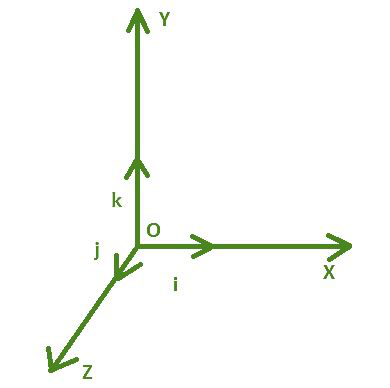Open in App
Not now

# Unit Vector Formula

• Last Updated : 26 Apr, 2022

Vectors are two-dimensional geometrical entities with position and orientation. A vector has two points: a launching point and an endpoint, which reflects the point’s final position. Adding, reduction, and multiplication are some of the arithmetic operations that can be performed on vectors. A unit vector is defined as a vector with a magnitude of one.### Unit Vector

The unit vector of a given vector is the one that can be obtained by dividing the former by its own magnitude. A unit vector is one whose magnitude is equal to one. It is depicted with a letter of the alphabet with an inverted V or cap on top of it. Unit vectors have a length of one. Unit vectors are commonly used to indicate a vector’s direction. The direction of a unit vector is the same as that of the provided vector, but its magnitude is one unit.

Formulawhere,

•denotes the vector ai + bj + ck
•denotes the magnitude of vector v as### Sample Problems

Problem 1. Find the unit vector of 2i + 4j + 5k.

Solution:

v = 2i + 4j + 5k= 3√5

Now,= (2/3√5) i + (4/3√5) j + (√5/3)k

Problem 2. Find the unit vector of 3i + 4j + 5k.

Solution:

v = 3i + 4j + 5k= 5√2

Now,= (3/5√2) i + (4/5√2) j + (1/√2)k

Problem 3. Find the unit vector of i + 2j + 2k.

Solution:

v =  i + 2j + 2k= 3

Now,= (1/3) i + (2/3) j + (2/3)k

Problem 4. Find the unit vector of the resultant vector of i + 3j +5k and -j – 3k.

Solution:

Resultant vector of i + 3j +5k and -j – 3k = (1+0)i + (3-1)j + (5-3)k

= i + 2j + 2k= 3

Now,= (1/3) i + (2/3) j + (2/3)k

Problem 5. Find the unit vector of 4i + 4j.

Solution:

v = 4i + 4j + 5k= 4√2

Now,= (1/√2) i + (1/√2) j

My Personal Notes arrow_drop_up
Related Articles